# Type C - Alphabet Codes / Code Words

With this type of question you are required to work out the code that has been used and to encode or decode a word.

You will be given a word and the coded version of this word. From this information you will need to work out the relationships between the letters of the word and the letters of the code. You will then either be asked to encode another word using the same method or decode a coded word to find the original.

## A B C D E F G H I J K L M N O P Q R S T U V W X Y Z

### The code for PLUM is QMVN. What is the code for DATE?

The strategy that I use to answer this type of question is to write the code directly below the word leaving enough space in between to add the rule. In this case,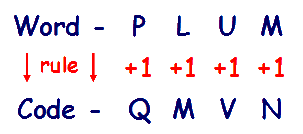By using the alphabet, you can see that the 1st letter of the word, P, becomes Q if you count on one letter, +1. The 2nd letter of the word, L, becomes M if you count on one letter, +1 and so on.

The rule for encoding a word is +1,+1,+1,+1
(you need to check each letter position)

We now know the coding rules - +1,+1,+1,+1 for each letter position.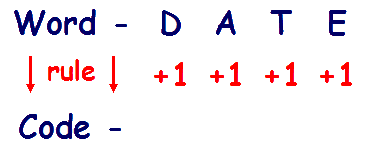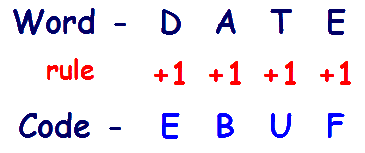Write out the word - D A T E with the rules directly below each letter.

It is now simply a case of encoding each letter in turn using the rule . D +1 is E, A +1 is B etc

Write the code letters directly below and you will have the code E B U F.

The code for DATE is EBUF.

### What does QFBS mean?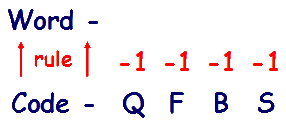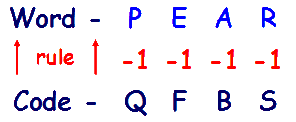Write out the code - Q F B S

Decoding is the opposite of encoding so to get the word from the code we need to reverse the rules, so +1 becomes -1. Write the rules directly above the code.

Now decode each letter in turn using the rules - working upwards this time. Q -1 is P, F -1 is E etc

Write the word letters directly above the code letters and you will have the word P E A R.

I find it easier to always have the word above the code and to work down to encode and up to decode. Care must be taken to remember to reverse the direction of the rules, especially as the rules can be a mixture of - and +. This is why writing it down in the same format is important.

Ensure that when working out the rules you check each position - the rules can follow a sequence in the same way that VR Type questions do. Some examples of coding rules are:

## Another form of VR Type C is the Mirror Image.

In these questions, the alphabet is mirrored.

##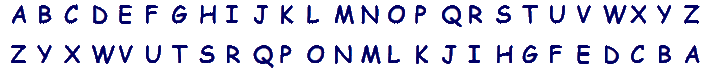Any letter is encoded (or decoded) into its corresponding mirror letter.
A becomes Z, B becomes Y, G becomes T and so on.

Another way to look at this is to divide the alphabet in two, between M and N and to count the same distance from the middle or ends.

## A B C D E F G H I J K L M | N O P Q R S T U V W X Y Z

L is -2 from the centre so it will code into O (+2 from the centre). D is 4 in from the left so it will code into W (4 in from the right)

It doesn't matter which method you use - I find the 1st method much quicker (you simply write the alphabet backwards underneath the normal alphabet)

This form of Type C can be hard to identify. If you are struggling to find a code using the normal method it is probably because it is a mirror image type! I get my students to remember the first 3 or 4 letter combinations, A-Z, B-Y, C-X and D-W and look for these combinations.